# Trinomials of the formwhereMost trinomials do not factor with integer values. However, it is always satisfying to find trinomials that do. Factoring helps us to solve equations efficiently, to graph relations easily and to develop mathematical arguments. All trinomials on this page do factor nicely.

A trinomial wherecan be tricky to factor. On this page, we think strategically through each problem to find the solution. Thinking strategically is often a preference – it is fast technique, it keeps you engaged with the problem.

There are a number of different approaches to factoring trinomials where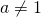. One of them is an algorithm (a set of steps) called decomposition. It might be initially tricky – however with some practice students can become quick at this technique. Another technique is a grid method: Factor Trinomial using a Grid Method

Here are some examples of thinking through a trinomial strategically.

Example 1: No constant termThe highest common factor of these two terms is.Example 2: There is a common factor between all three termsFirst we notice that 2 divides all three terms. Let’s factor it out:Notice we cannot ‘divide’ by two, as we have an expression, not an equation. When manipulating an expression, we must not change its value. We can’t halve it.

Now, within the brackets, we have a trinomial withand so we simply find a factor pair that multiplies to 4 and adds to -5. I’m thinking -4 and -1 will do it!Example 3: Whereis a prime number.Now, when we lay out the brackets, the only integer pair that multiplies to the leading term isand. So we begin with that: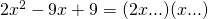Next, we need to choose between factor pairs 9, 1 (or 1, 9) and 3, 3 to make the product c = 9. We also note that they both have to be negative. (why?) We guess and check until it works out.nopeagain, nolovely. Done!

A prime number as a coefficient ofreduces the number of guesses we need to perform.Example 4: Whereis a prime number (or 1)Now, when we lay out the brackets, the only integer pair that multiplies to the constant termisand. So we begin with that:Now we need to choose between factor pairs 4, 1 (or 1, 4) or 2,2 for the leading coefficient. Let’s try them out:No, this give +3x in the middle.Nice, this does it.

After only two guesses, we reach our solution: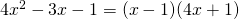Although, for reasons that might seem absolutely random, it is traditional to write it with the larger value first: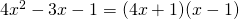Example 5: Where neithernoris a prime numberIn this example we might need to do extensive scribbling in the margin. We must also remember that one of the primary reasons we factor is for speed and efficiency. If we do not find a factorization soon enough, chances are high (when you’re not on this page) your trinomial does not factor nicely. If we are trying to factor in order to be able to solve an equation or to graph, and if it is taking too long, we use a different technique for solution – either ‘completing the square’ or ‘the quadratic formula’. These are taught in a different course.

On our side for this example is the fact that it is a ‘+, +’ trinomial. Our search is limited to just positive values.

Factor pairs for 10 are: 1, 10; also 2, 5 and vice versa for each.

Factor pairs for 9 are: 1, 9 and vice versa; and  3,3.

Let’s begin our checking out the options:No, this givesin the middle…. we really only have to examine the middle terms.No, this gives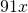in the middle.Great! We havein the middle. So we have our solution: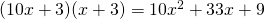Here is an applet to practice. Fill in the two brackets, multiply out mentally (or on paper) before you press enter.

Worksheet & Solutions: Factor Trinomial a not equal to 1

Done here! Go back to the Factors and Products Menu.